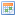ENQUIRY WINTER PACKAGES

ENQUIRY WINTER PACKAGES

•  1Select a package > 2Accommodation > 3Address
• / /• / /• / /• / /• / /• / /• / /• / /• / /• / /• / /• / /• / /• / /• / /• / /• / /• / /• / /• / /• / /• / /• / /• / /• / /• / /• / /• / /• / /• / /• / /• / /• / /• / /